Latest Banking jobs   »   IBPS quant

# Quantitative Aptitude Quiz For IBPS Clerk Mains 2023-19th-September

Direction (1-5): The following line graph shows the distribution of total number of teachers in five (A, B, C, D and E) different schools. Read the following line graph carefully and answer the questions given below.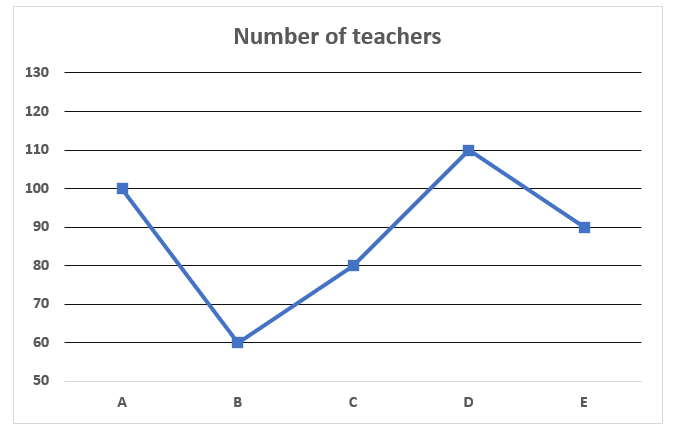Q1. If in school F, the number of teachers are 50% more than the average number of teachers in school B and C together, then find the number of teachers in school F.
(a)140
(b)115
(c)105
(d)100
(e)120
Q2. Find the respective ratio of number of teachers in school A to number of teachers in D & E together?
(a) 1:2
(b)5:4
(c)1:1
(d)2:3
(e)3:2
Q3. Find the number of teachers in school A is what percent more/less than the number of teachers in school C?
(a)10%
(b)25%
(c)30%
(d)50%
(e)35%
Q4. If the number of male teachers in school D are 30 less than the female teachers, then find the total number of female teachers in school D?
(a)85
(b)65
(c)60
(d)70
(e)75
Q5. Find the difference between the average number of teachers in school A, B & C and that in school C & E.
(a)15
(b)25
(c)10
(d)20
(e)5
Direction (6-10): The following bar graph shows the total number of questions attempted and total questions answered correctly by the five (P, Q, R, S and T) different students. Read the following bar graph carefully and answer the questions given below.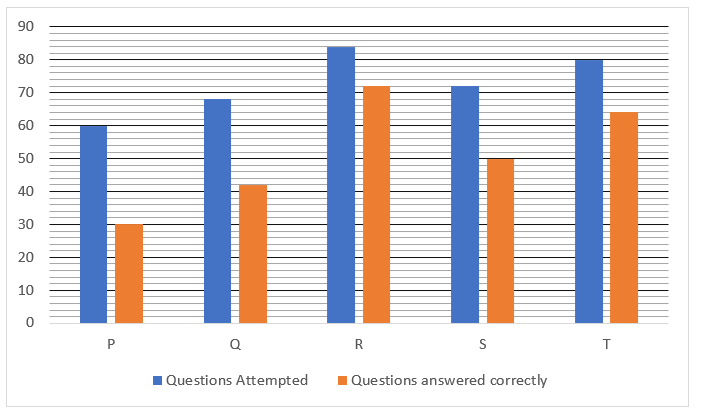Q6. Find the average number of questions answered correctly by P & R.
(a)44
(b)51
(c)54
(d)48
(e)58
Q7. Find the ratio of number of questions answered wrongly by P & T together to number of questions answered wrongly by R?
(a)21:17
(b)8:23
(c) 23:6
(d)17:21
(e)21:20
Q8. Find the number of questions answered wrongly by Q is what percent of the number of questions answered correctly by S?
(a)125%
(b)75%
(c)100%
(d)52%
(e)50%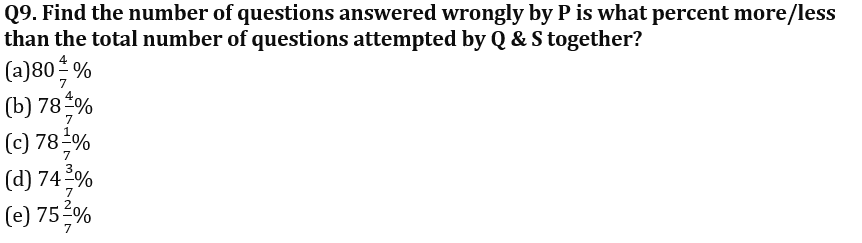Q10. If another student A had attempted twice the number of questions attempted by S and the number of questions answered wrongly by A are 40 less than the number of questions attempted by R, then find the number of questions answered correctly by A?
(a)110
(b)80
(c)90
(d)100
(e)120

Solutions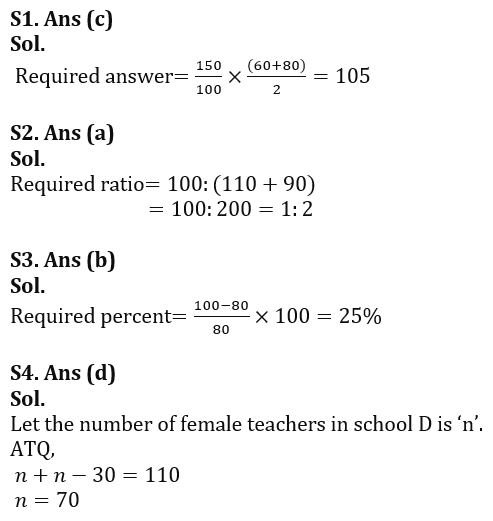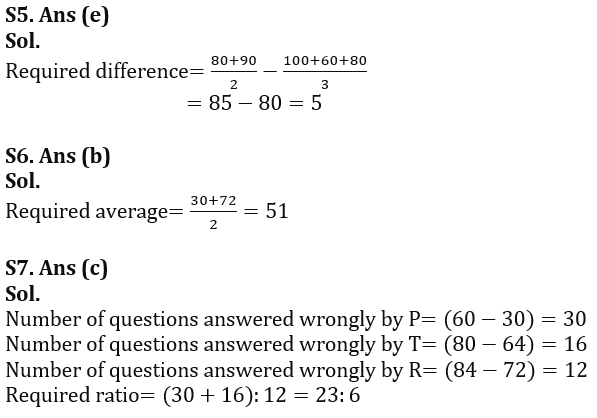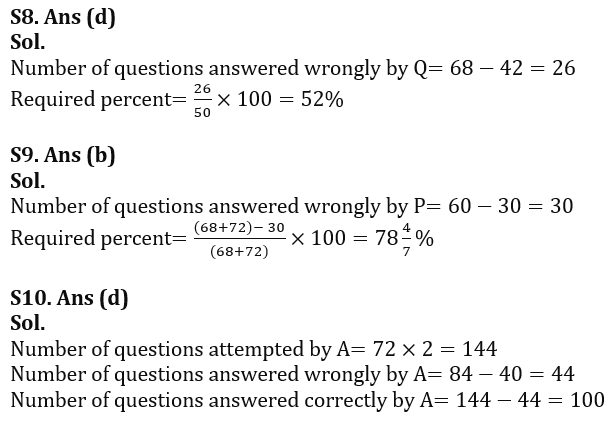## FAQs

### When is the IBPS Clerk Mains exam scheduled for?

The IBPS Clerk Mains exam is scheduled for 7 October 2023.

#### Congratulations!Union Budget 2023-24: Free PDF